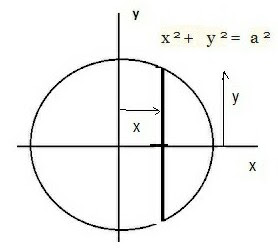## Wednesday, November 23, 2022

### Using Integration to Find the Center of Mass of a Solid Object

Obtaining the center of mass for a system or object is an important one in general physics.  Indeed, we've already seen the technique for obtaining the center of mass of a binary star system with the line of centers treated as a 1-dimensional problem, e.g.

Revisiting Binary Stars

From calculus-based general physics (or classical mechanics) , the general equations for the center of mass of a 3D solid as a whole are given by:

x  =     ò x dm /   ò dm,    y  =     ò y dm / ò dm,

z  =     ò z dm /   ò dm

The derivation, which will not be done here, is generally by adding the moments (for basic principles of moments see:

Of all the concentrated masses in all the volume elements  V  and taking the limit as the  V 's approach 0.

An interesting introductory problem for a planar 3D object, say a wafer-thin, homogeneous triangular plate with height h and base dimension b, is laid out as shown in the sketch of mind below:

The basic procedure entails dividing the triangle into strips of width dy parallel to the x-axis. The mass of the strip then is approximately:

dm =  d dA

Where dA =    ℓ  dy

With     the width of the triangle at the distance y above its base. Using similar triangles we get:

/ b  =  (h - y) / h

Then:

=  b/h  (h - y)

So the element of mass will be expressed:

dm =   (d )   b/h  (h - y) dy

For the entire plate we can then write for the y-coordinate of the center of mass:

y  =     ò y dm /   ò dm

=  ò 0   y db/h  (h - y) dy/  ò 0   b/h  (h - y) dy

= h  / 3

Thus, the center of mass of the uniform triangular plate lies at a distance one third of the way toward the opposite vertex.

An interesting introductory problem  for a 3D object is to find the position of the center of mass for a uniform solid hemisphere – as shown in the graphic below.This is take to have a radius a, and a uniform density,r,  and we note the center of the plane face of the hemisphere is the origin.

The x-axis is perpendicular to this plane strip or face.  By symmetry the center of mass will lie on the x-axis.  We begin by considering an elemental strip of thickness  d x with its plane face perpendicular to the x-axis and distance x from the origin.  If its radius is given by y the volume of the disk is:

V  =  p y 2  d x

With the element of mass given by:

d m  =  p y 2  d x r

And so we can write for the x -coordinate of the center of mass for the elemental disk:

x   ~  å a  x=o   x p y 2 r d x/  å d m

Or:

~   å a  x=o   x p y 2  r d x/  Total mass

In the limit as  d x  -> 0

x =  ò 0   x p y 2 r dx

And:   2 p a 3 r/ 3 =

p r   ò 0   x y 2 dx

x 2 a 3 / 3 =

ò 0   x (a 2 -   2) dx

=   ò 0   (xa 2 -   3)  dx

= [ a 2 x 2 /2  -   x4  /4] 0

= [ a 4 /2  -   a4  /4]-  0

=  a4  /4

x=   a4  /4    ( 3/2 a 3 )  =   3 a /8

Suggested Problems:

1) A straight, thin rod PQ of length 2a of constant cross sectional area A is of variable density, r.
Given r  = ro (1 + 2x 2 / a 2)  where x is the distance along the rod from the end of P and ro  is a constant.  Find the position of the center of mass.

2) Find the center of mass of a solid hemisphere of radius r if the density at any point P is proportional to the distance of P from the base of the hemisphere.  (Hint:  Add another lower latitutde layer to the upper latitude circle below to form a thin slice of thickness dy.  Then imagine the whole hemisphere cut into slices of thickness dy, i.e. by planes perpendicular to the y-axis)